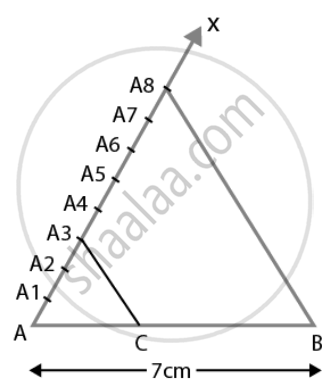# Draw a line segment of length 7 cm. Find a point P on it which divides it in the ratio 3:5 - Mathematics

Diagram
Sum

Draw a line segment of length 7 cm. Find a point P on it which divides it in the ratio 3:5

#### SolutionSteps of construction:

1. Draw a line segment, AB = 7 cm.

2. Draw a ray, AX, making an acute angle downward with AB.

3. Mark the points A1, A2, A… A8 on AX.

4. Mark the points such that AA1 = A1A2 = A2A3 = ….., A7A8.

5. Join BA8.

6. Draw a line parallel to BA8 through the point A3, to meet AB on P.

Hence AP: PB = 3:5

Concept: Division of a Line Segment
Is there an error in this question or solution?

#### APPEARS IN

NCERT Mathematics Exemplar Class 10
Chapter 10 Construction
Exercise 10.3 | Q 1 | Page 116
Share